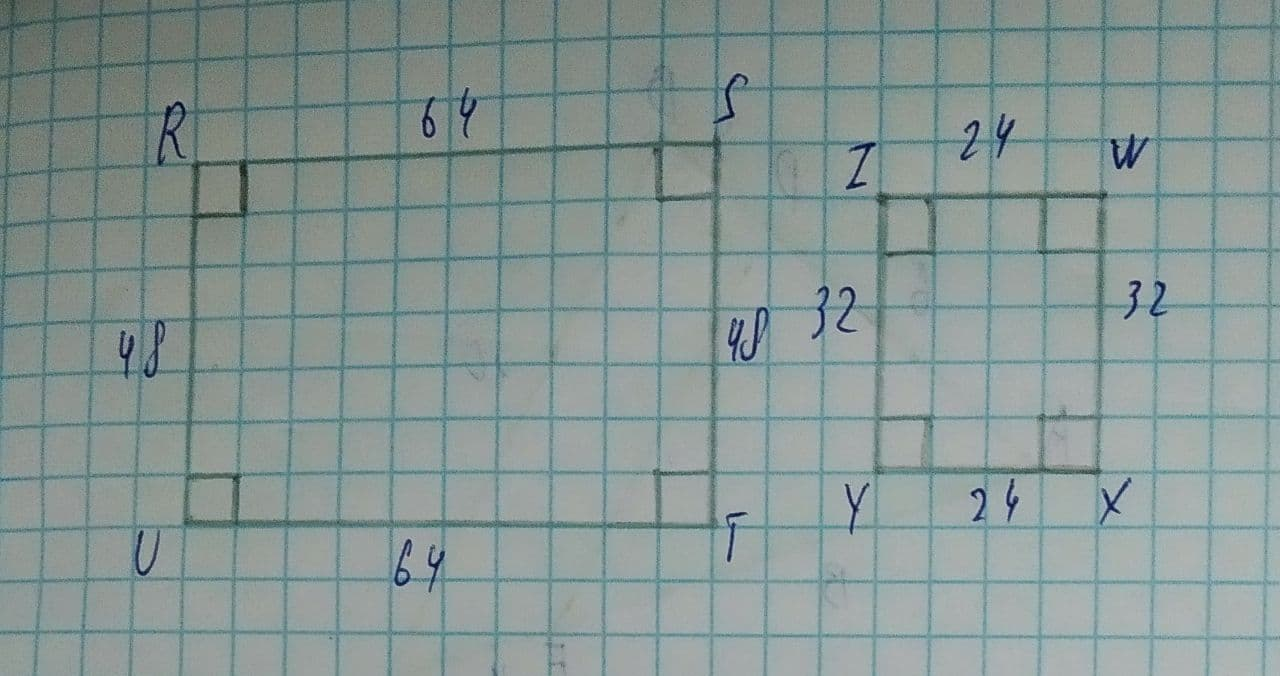# Determine whether the polygons are similar. If they are, write a similarity statement and find the scale factor.pedzenekO 2021-08-08 Answered
Determine whether the polygons are similar. If they are, write a similarity statement and find the scale factor.You can still ask an expert for help

• Questions are typically answered in as fast as 30 minutes

Solve your problem for the price of one coffee

• Math expert for every subject
• Pay only if we can solve itMayme
Step 1
The quadrilateral, UTSR has opposite sides equal and they are 48 & 64
So, the adjacent sides are in the ratio, 48:64 = 3:4
In the quadrilateral, YXWZ, the opposite sides are equal as well and the adjacent sides are in the ratio, 24:32 = 3:4
So, they must be similar.
Step 2
The rule for similarity is, S-S-S-S and the scale factor is, 2 or ($\frac{1}{2}$)
And, we have, $UTSR\sim XWZY$